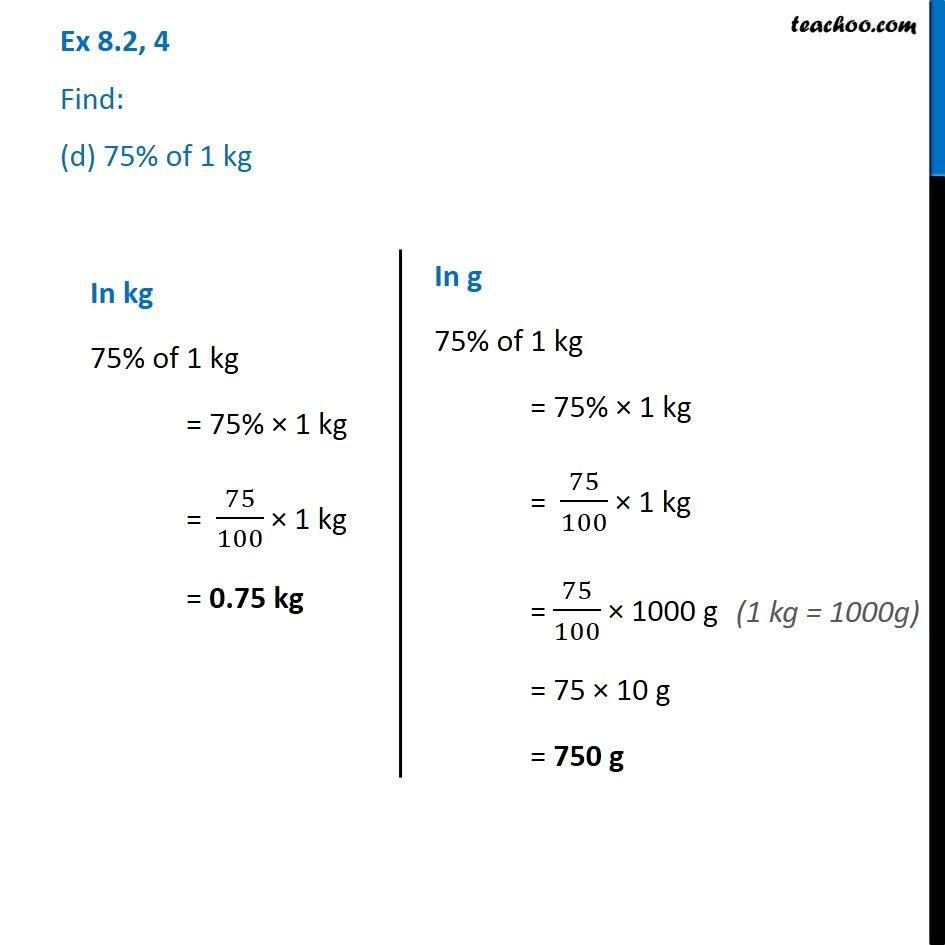Ex 8.2

Chapter 8 Class 7 Comparing Quantities
Serial order wiseGet live Maths 1-on-1 Classs - Class 6 to 12

### Transcript

Ex 8.2, 4 Find: (d) 75% of 1 kg In kg 75% of 1 kg = 75% × 1 kg = 75/100 × 1 kg = 0.75 kg In g 75% of 1 kg = 75% × 1 kg = 75/100 × 1 kg = 75/100 × 1000 g = 75 × 10 g = 750 g (1 kg = 1000g)